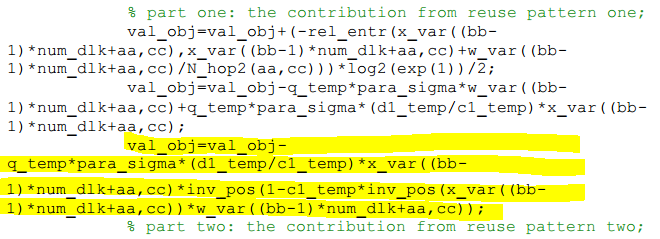# How to present my convex optimization in a CVX-compliant way

Hello,

I am new to CVX optimization toolbox. Recently, I am trying to solve a convex optimization via CVX in MATLAB, but I get an error stated as follows,

“Disciplined convex programming error:
Cannot perform the operation: {convex} .* {real affine}”

In the following, I give more details to my problem,

Finally, the above error is incurred when expressing the following segment within the objective function,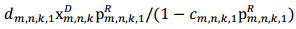by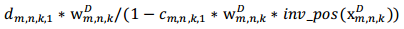So, I just wonder if it is possible for me to express it in a CVX-compliant way, and if yes, how can I make the adjustment.

Kind regards,
Allan

You haven’t offered up any code so it’s unlikely we will be able to help you. If you do post code, please make sure to isolate exactly the line that is causing the problem, and make sure we know which quantities are declared as variables.

Hello, Ph.D. Grant,

Thank you for you timely reply. In this message, I would like to make some complement to my original problem, and then attach my MATLAB code for a better trouble-shooting.

After introducing the two auxiliary variables, i.e.,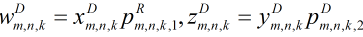,
the original problem in (1) is transferred into (3) below (please forgive my rashness, I miss a linear constraint in the original form, i.e., (3-b), though it will not affect the concavity of the problem),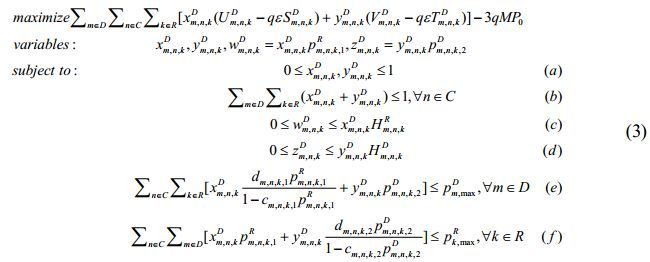The corresponding MATLAB code:

``````Error using cvx/times (line 173)
Disciplined convex programming error:
Cannot perform the operation: {convex} .* {real affine}

Error in cvx/mtimes (line 36)
z = feval( oper, x, y );

Error in EEMR_sub9>Fun_obj (line 87)
val_obj=val_obj-q_temp*para_sigma*(d1_temp/c1_temp)*x_var((bb-1)*num_dlk+aa,cc)*inv_pos(1-c1_temp*inv_pos(x_var((bb-1)*num_dlk+aa,cc))*w_var((bb-1)*num_dlk+aa,cc));``````

You haven’t show us what `Fun_obj` or is; nor do we know exactly where the error is occurring.

Hello, Ph.D. Grant,